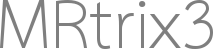# "Effective DOF" of SIFT/SIFT2 Models

Hello MRtrixperts,
I’ve been trying to do model comparisons between (SIFTed) tractograms to determine which fits the diffusion data better (using after_diff_fixel.msf). Usually, I’m trying to compare some full tractogram to a restricted tractogram (i.e., with some streamlines of interest removed), as in the “virtual lesioning” approach of Pestelli et al., 2014. What I’m getting stuck on is the “effective degrees of freedom” of each SIFT(2) model.

For a typical F-test (nested model comparison), the degrees of freedom are (p 2 − p 1, np 2), where n is the number of data points (fixels) and p is the # of parameters (# of streamlines) in each model. But here, p2 > n because there are more streamlines than fixels and the streamlines are correlated predictors. So then, what are the effective degrees of freedom of a SIFT(2) model?

The regularized regression literature suggests using the trace of the “hat” matrix (the projection matrix). Is that something that would be feasible to get out of SIFT/SIFT2?

Any help would be greatly appreciated! My apologies for the arcane questionJohn

… Yikes.While it’s probably feasible to do something here, it’s likely best taken offline. There’s a fair bit of math to go over here, and it’s certainly not something that’s going to be achieved without writing a considerable amount of C++ code: I went out of my way to avoid explicitly dealing with covariances in both algorithms due to the size of the system, but obtaining the projection matrix requires such, which means going in to the guts of the sparse RAM representation of the streamline -> fixel matrix.

Rob